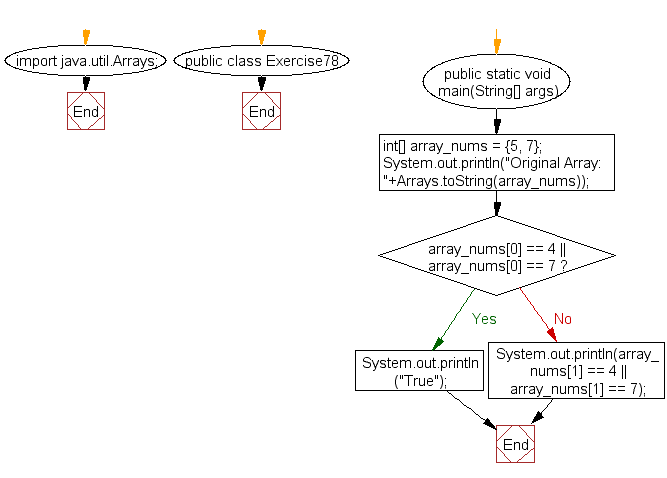﻿ Java: An array of integers of length 2 contains a 4 or a 7# Java Exercises: Test that a given array of integers of length 2 contains a 4 or a 7

## Java Basic: Exercise-78 with Solution

Write a Java program to test that a given array of integers of length 2 contains a 4 or a 7.

Sample Solution:

Java Code:

``````import java.util.Arrays;
public class Exercise78 {
public static void main(String[] args)
{
int[] array_nums = {5, 7};
System.out.println("Original Array: "+Arrays.toString(array_nums));
if(array_nums == 4 || array_nums == 7)
System.out.println("True");
else
System.out.println(array_nums == 4 || array_nums == 7);

}
}
```
```

Sample Output:

```Original Array: [5, 7]
true
```

Pictorial Presentation:Flowchart:Java Code Editor:

What is the difficulty level of this exercise?

Test your Programming skills with w3resource's quiz.

﻿

## Java: Tips of the Day

countOccurrences

Counts the occurrences of a value in an array.

Use Arrays.stream().filter().count() to count total number of values that equals the specified value.

```public static long countOccurrences(int[] numbers, int value) {
return Arrays.stream(numbers)
.filter(number -> number == value)
.count();
}
```

Ref: https://bit.ly/3kCAgLb